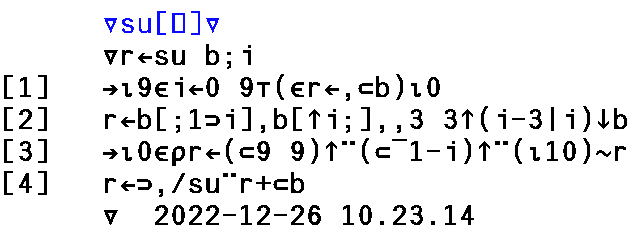﻿ APL2 Sudoku solver

APL2 Sudoku solver

This is an APL (actually APL2) function to solve a Sudoku puzzle, developed using MicroAPL APLX ver 5.1.0.  "Solved" simply means a 1-9 could be legally placed in all empty squares.

The puzzle to solve is the argument to the function -- it should be a 9 x 9 numeric matrix with a 0 to indicate an empty square.  The input puzzle is not checked in any way... it is assumed to be valid.

The function requires the index origin is set to zero.  It uses a brute force method (try all possible numbers) so it's very slow!

The result is a nested vector.  The rho of the result is the number of solutions found (0..n) -- this should be 1 for a legal puzzle.  The content of the vector are the 9 x 9 numeric matrix solutions.

r←su b;i
→⍳9∊i←0 9⊤(∊r←,⊂b)⍳0
r←b[;1⊃i],b[↑i;],,3 3↑(i-3|i)↓b
→⍳0∊⍴r←(⊂9 9)↑¨(⊂¯1-i)↑¨(⍳10)~r
r←⊃,/su¨r+⊂b